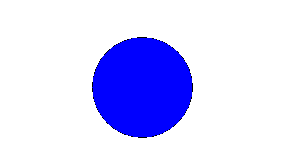# Java program to draw a circle

In this java program, we are going to learn how to draw a circle using 'Shape' class? Here, we are using 'java.awt.*' related packages, classes to draw a circle.
Submitted by IncludeHelp, on November 19, 2017

By using java program, we have to draw a circle.

```import java.awt.*;
import java.awt.event.*;
import java.awt.geom.*;

public class DrawCircle extends Frame
{
// input the value for circle and square.
Shape circle=new Ellipse2D.Float(100.0f,100.0f,100.0f,100.0f);

// class paint to fill color in circle.
public void paint(Graphics g)
{
Graphics2D ga=(Graphics2D)g;
ga.draw(circle);
ga.setPaint(Color.blue);
ga.fill(circle);
}

public static void main(String args[])
{
// create a frame object for circle.
Frame frame=new DrawCircle();
{
public void windowClosing(WindowEvent we)
{
System.exit(0);
}
});
// circle coordinates.
frame.setSize(300, 250);
frame.setVisible(true);
}
}
```

Output